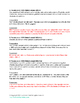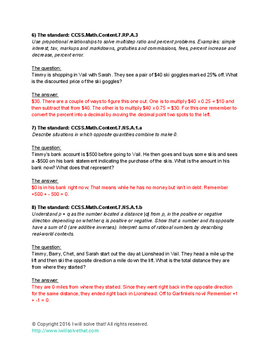# Math: The Book of Timmy (7th Grade Edition) With More Bacon!Subject
Resource Type
File Type

PDF

(315 KB|19 pages)
Product Rating
Standards
• Product Description
• StandardsNEW
In this edition of The Book of Timmy, Timmy tackles the 7th grade math Common Core standards with 36 "bell ringer" problems covering every Common Core math standard in 7th grade. Each problem has the standard included along with a detailed explanation as to the answer to each problem.
Use proportional relationships to solve multistep ratio and percent problems. Examples: simple interest, tax, markups and markdowns, gratuities and commissions, fees, percent increase and decrease, percent error.
Explain what a point (𝘹, 𝘺) on the graph of a proportional relationship means in terms of the situation, with special attention to the points (0, 0) and (1, 𝘳) where 𝘳 is the unit rate.
Represent proportional relationships by equations. For example, if total cost 𝘵 is proportional to the number 𝘯 of items purchased at a constant price 𝘱, the relationship between the total cost and the number of items can be expressed as 𝘵 = 𝘱𝘯.
Identify the constant of proportionality (unit rate) in tables, graphs, equations, diagrams, and verbal descriptions of proportional relationships.
Decide whether two quantities are in a proportional relationship, e.g., by testing for equivalent ratios in a table or graphing on a coordinate plane and observing whether the graph is a straight line through the origin.
Total Pages
19 pages
Included
Teaching Duration
N/A
Report this Resource to TpT
Reported resources will be reviewed by our team. Report this resource to let us know if this resource violates TpT’s content guidelines.